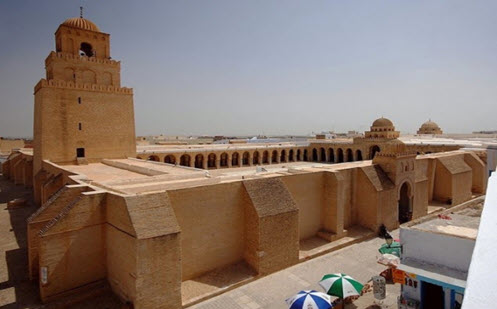Go to website

# More rectangle ratios

This prompt is made up of a square and rectangle, which together form a large rectangle. The ratio of the length to the width of the large rectangle equals the ratio of length to width of the smaller rectangle. The ratio in the prompt is satisfied by the proportions of the golden rectangle whose length and width are in the golden ratio (φ or phi). The resource includes classroom examples, teacher notes and guided questions.

Year level(s) Year 8, Year 9
Audience Teacher
Teaching strategies and pedagogical approaches Collaborative learning, Differentiated teaching, Explicit teaching, Feedback, Mathematics investigation
Keywords square, rectangle, area, ratio

## Curriculum alignment

Curriculum connections Critical and creative thinking, Numeracy
Strand and focus Algebra, Number, Measurement, Build understanding, Apply understanding
Topics Area, volume and surface area, Proportion, rates and ratios
AC: Mathematics (V9.0) content descriptions
AC9M8M05
Recognise and use rates to solve problems involving the comparison of 2 related quantities of different units of measure

AC9M9M05
Use mathematical modelling to solve practical problems involving direct proportion, rates, ratio and scale, including financial contexts; formulate the problems and interpret solutions in terms of the situation; evaluate the model and report methods and findings

Numeracy progression Number patterns and algebraic thinking (P8)
Proportional thinking (P5, P7)
Understanding geometric properties (P7)
Understanding money (P9)